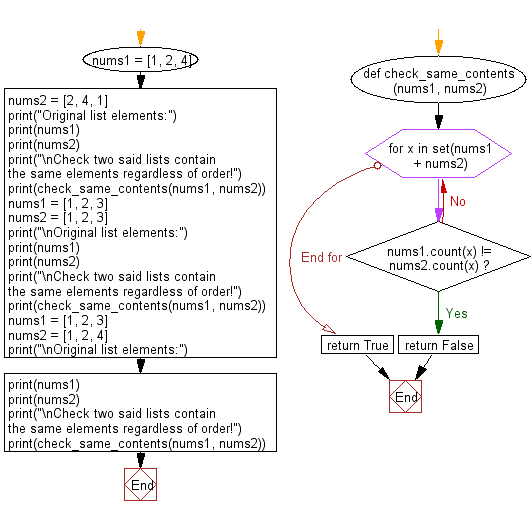﻿ Python: Check if two lists contain the same elements regardless of order - w3resource# Python: Check if two lists contain the same elements regardless of order

## Python List: Exercise - 257 with Solution

Write a Python program to check if two given lists contain the same elements regardless of order.

• Use set() on the combination of both lists to find the unique values.
• Iterate over them with a for loop comparing the count() of each unique value in each list.
• Return False if the counts do not match for any element, True otherwise.

Sample Solution:

Python Code:

``````def check_same_contents(nums1, nums2):
for x in set(nums1 + nums2):
if nums1.count(x) != nums2.count(x):
return False
return True
nums1 = [1, 2, 4]
nums2 = [2, 4, 1]
print("Original list elements:")
print(nums1)
print(nums2)
print("\nCheck two said lists contain the same elements regardless of order!")
print(check_same_contents(nums1, nums2))
nums1 = [1, 2, 3]
nums2 = [1, 2, 3]
print("\nOriginal list elements:")
print(nums1)
print(nums2)
print("\nCheck two said lists contain the same elements regardless of order!")
print(check_same_contents(nums1, nums2))
nums1 = [1, 2, 3]
nums2 = [1, 2, 4]
print("\nOriginal list elements:")
print(nums1)
print(nums2)
print("\nCheck two said lists contain the same elements regardless of order!")
print(check_same_contents(nums1, nums2))
```
```

Sample Output:

```Original list elements:
[1, 2, 4]
[2, 4, 1]

Check two said lists contain the same elements regardless of order!
True

Original list elements:
[1, 2, 3]
[1, 2, 3]

Check two said lists contain the same elements regardless of order!
True

Original list elements:
[1, 2, 3]
[1, 2, 4]

Check two said lists contain the same elements regardless of order!
False
```

Flowchart:## Visualize Python code execution:

The following tool visualize what the computer is doing step-by-step as it executes the said program:

Python Code Editor:

Have another way to solve this solution? Contribute your code (and comments) through Disqus.

What is the difficulty level of this exercise?

Test your Python skills with w3resource's quiz

﻿

## Python: Tips of the Day

Floor Division:

When we speak of division we normally mean (/) float division operator, this will give a precise result in float format with decimals.

For a rounded integer result there is (//) floor division operator in Python. Floor division will only give integer results that are round numbers.

```print(1000 // 300)
print(1000 / 300)```

Output:

```3
3.3333333333333335```